## 一、多态的定义

• 派生类对象的地址可以赋值给基类指针。对于通过基类指针调用基类和派生类中都有的同名、同参数表的虚函数的语句，编译时并不确定要执行的是基类还是派生类的虚函数；而当程序运行到该语句时，如果基类指针指向的是一个基类对象，则基类的虚函数被调用，如果基类指针指向的是一个派生类对象，则派生类的虚函数被调用。这种机制就叫作“多态（polymorphism）”。多态可以简单地理解为同一条函数调用语句能调用不同的函数；或者说，对不同对象发送同一消息，使得不同对象有各自不同的行为。

• 所谓“虚函数”，就是在声明时前面加了 virtual 关键字的成员函数。virtual 关键字只在类定义中的成员函数声明处使用，不能在类外部写成员函数体时使用。静态成员函数不能是虚函数。包含虚函数的类称为“多态类”。

*例如以下程序：

#include <iostream>
using namespace std;
class A
{
public:
virtual void Print() { cout << "A::Print" << endl; }
};
class B : public A
{
public:
virtual void Print() { cout << "B::Print" << endl; }
};
class D : public A
{
public:
virtual void Print() { cout << "D::Print" << endl; }
};
class E : public B
{
virtual void Print() { cout << "E::Print" << endl; }
};
int main()
{
A  a; B b; D d; E e;
A *pa = &a;  B *pb = &b;
pa->Print();    //多态， a.Print()被调用，输出：A::Print
pa = pb;        //基类指针pa指向派生类对象b
pa->Print();  //b.Print()被调用，输出：B::Print
pa = &d;       //基类指针pa指向派生类对象d
pa->Print();  //多态， d. Print ()被调用,输出：D::Print
pa = &e;       //基类指针pa指向派生类对象d
pa->Print();  //多态， e.Print () 被调用,输出：E::Print
return 0;
}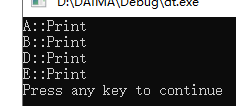## 二、通过基类引用实现多态

• 通过基类的引用调用虚函数的语句也是多态的。即，通过基类的引用调用基类和派生类中同名、同参数表的虚函数时，若其引用的是一个基类的对象，则被调用是基类的虚函数；若其引用的是一个派生类的对象，则被调用的是派生类的虚函数。
#include <iostream>
using namespace std;
class A
{
public:
virtual void Print() { cout << "A::Print" << endl; }
};
class B : public A
{
public:
virtual void Print() { cout << "B::Print" << endl; }
};
void Printlnfo(A & r)
{
r.Print();  //多态，调用哪个Print，取决于r引用了哪个类的对象
}
int main()
{
A a; B b;
Printlnfo(a);  //输出 A::Print
Printlnfo(b);  //输出 B::Print
return 0;
}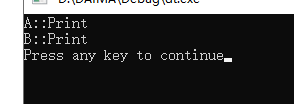## 三、静态多态和动态多态

### 1.静态多态

#include<iostream>
using namespace std;
int Add(int left, int right)
{
return left + right;
}
double Add(double left, int right)
{
return left + right;
}

int main()
{
return 0;
}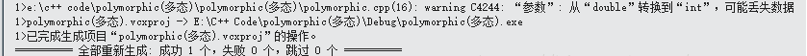### 2.动态多态

#include<iostream>
using namespace std;
class TakeBus
{
public:
void TakeBusToSubway()
{
cout << "go to Subway--->please take bus of 318" << endl;
}
void TakeBusToStation()
{
cout << "go to Station--->pelase Take Bus of 306 or 915" << endl;
}
};
//知道了去哪要做什么车可不行，我们还得知道有没有这个车
class Bus
{
public:
virtual void TakeBusToSomewhere(TakeBus& tb) = 0;  //？？？为什么要等于0
};

class Subway :public Bus
{
public:
virtual void TakeBusToSomewhere(TakeBus& tb)
{
tb.TakeBusToSubway();
}
};
class Station :public Bus
{
public:
virtual void TakeBusToSomewhere(TakeBus& tb)
{
tb.TakeBusToStation();
}
};

int main()
{
TakeBus tb;
Bus* b = NULL;
//假设有十辆公交车，如果是奇数就是去地铁口的，反之就是去火车站的
for (int i = 1; i <= 10; ++i)
{
if ((rand() % i) & 1)
b = new Subway;
else
b = new Station;
}
b->TakeBusToSomewhere(tb);
delete b;
return 0;
}


#include<iostream>
using namespace std;
class Base
{
public:
virtual void Funtest1(int i)
{
cout << "Base::Funtest1()" << endl;
}
void Funtest2(int i)
{
cout << "Base::Funtest2()" << endl;
}
};
class Drived :public Base
{
virtual void Funtest1(int i)
{
cout << "Drived::Fubtest1()" << endl;
}
void Funtest2(int i)
{
cout << "Drived::Fubtest2()" << endl;
}
};
void TestVirtual(Base& b)
{
b.Funtest1(1);
b.Funtest2(2);
}
int main()
{
Base b;
Drived d;
TestVirtual(b);
TestVirtual(d);
return 0;
}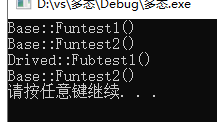*动态多态的条件：
*基类中必须包含虚函数，并且派生类中一定要对基类中的虚函数进行重写。
*通过基类对象的指针或者引用调用虚函数。

（a）基类中将被重写的函数必须为虚函数（上面的检测用例已经证实过了）
（b）基类和派生类中虚函数的原型必须保持一致（返回值类型，函数名称以及参数列表），协变和析构函数（基类和派生类的析构函数是不一样的）除外
（c）访问限定符可以不同

## 四、多态的实现原理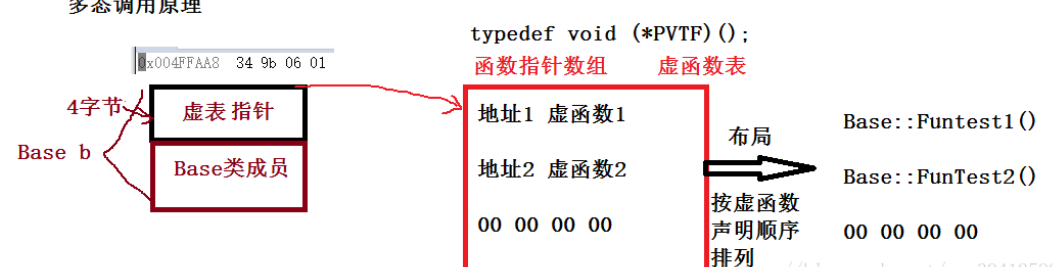## 五、多态的缺陷

• 降低了程序运行效率（多态需要去找虚表的地址）

• 空间浪费